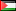##### Numerical Simulation of The Steady State Heat Conduction Equation
Publication Type
Original research
Authors
• Naji Qatanani

The main concern in this article is the numerical realization of the steady state heat conduction taking place in a three-dimensional enclosure geometries. For that purpose, we have derived the integral equation of heat conduction from the original boundary value problem using the weighted residual method. For the descritization of the conduction integral equation we have used the boundary element method based on the Galerkin-Bubnov scheme. The system of linear equations has been solved by the conjugate gradient- method with preconditioning. To demonstrate the high efficiency of this method a numerical experiment has been constucted for a three- dimensional simple pipe.

Journal
Title
International Journal of Mathematical Modeling, Simulation and Applications
Publisher
--
Publisher Country
PalestinePublication Type
Both (Printed and Online)
Volume
--
Year
2012
Pages
--